Students can download Maths Chapter 6 Trigonometry Ex 6.2 Questions and Answers, Notes, Samacheer Kalvi 10th Maths Guide Pdf helps you to revise the complete Tamilnadu State Board New Syllabus, helps students complete homework assignments and to score high marks in board exams.

## Tamilnadu Samacheer Kalvi 10th Maths Solutions Chapter 6 Trigonometry Ex 6.2

Question 1.
Find the angle of elevation of the top of a tower from a point on the ground, which is 30 m away from the foot of a tower of height 10 $$\sqrt { 3 }$$ m.
Height of the tower (AC) = 10 $$\sqrt { 3 }$$ m
Distance between the base of the tower and point of observation (AB) = 30 m
Let the angle of elevation ∠ABC be θ
In the right ∆ ABC, tan θ = $$\frac { AC }{ AB }$$
= $$\frac{10 \sqrt{3}}{30}=\frac{\sqrt{3}}{3}$$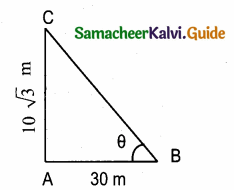tan θ = $$\frac{1}{\sqrt{3}}$$ = tan 30°
∴ Angle of inclination is 30°Question 2.
A road is flanked on either side by continuous rows of houses of height 4$$\sqrt { 3 }$$ m with no space in between them. A pedestrian is standing on the median of the road facing a row house. The angle of elevation from the pedestrian to the top of the house is 30° . Find the width of the road.
Let the mid point of the road AB is “P” (PA = PB)
Height of the home = 4$$\sqrt { 3 }$$ m
Let the distance between the pedestrian and the house be “x”
In the right ∆ APD, tan 30° = $$\frac { AD }{ AP }$$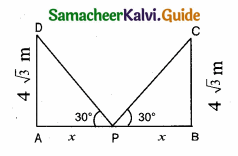$$\frac{1}{\sqrt{3}}=\frac{4 \sqrt{3}}{x}$$
x = 4 $$\sqrt { 3 }$$ × $$\sqrt { 3 }$$ = 12 m
∴ Width of the road = PA + PB
= 12 + 12
= 24 m

Question 3.
To a man standing outside his house, the angles of elevation of the top and bottom of a window are 60° and 45° respectively. If the height of the man is 180 cm and if he is 5 m away from the wall, what is the height of the window? ($$\sqrt { 3 }$$ = 1.732)
Let the height of the window FE be “h” m
Let FC be “x” m
∴ EC = (h + x) m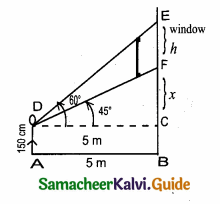In the right ∆ CDF, tan 45° = $$\frac { CE }{ CD }$$
1 = $$\frac { x }{ 5 }$$ ⇒ x = 5
In the right ∆ CDE, tan 60° = $$\frac { CE }{ CD }$$
$$\sqrt { 3 }$$ = $$\frac { x+h }{ 5 }$$ ⇒ x + h = 5$$\sqrt { 3 }$$
5 + h = 5 $$\sqrt { 3 }$$ (substitute the value of x)
h = 5 $$\sqrt { 3 }$$ – 5 = 5 × 1.732 – 5 = 8. 66 – 5 = 3.66
∴ Height of the window = 3.66 mQuestion 4.
A statue 1.6 m tall stands on the top of a pedestal. From a point on the ground, the angle of elevation of the top of the statue is 60° and from the same point the angle of elevation of the top of the pedestal is 40° . Find the height of the pedestal.
(tan 40° = 0.8391, $$\sqrt { 3 }$$ = 1.732)
Height of the statue = 1.6 m
Let the height of the pedestal be “h”
Let AB be x
In the right ∆ ABD, tan 60° = $$\frac { AD }{ AB }$$
$$\sqrt { 3 }$$ = $$\frac { h+1.6 }{ x }$$
x = $$\frac{h+1.6}{\sqrt{3}}$$ ……..(1)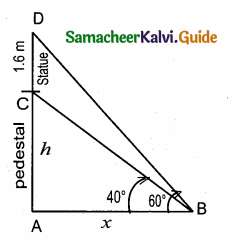In the right ∆ ABC, tan 40° = $$\frac { AC }{ AB }$$
0.8391 = $$\frac { h }{ x }$$
x = $$\frac { h }{ 0.8391 }$$
Substitute the value of x in (1)
$$\frac{h}{0.8391}=\frac{h+1.6}{\sqrt{3}}$$
(h + 1.6) 0.8391 = $$\sqrt { 3 }$$ h
0.8391 h + 1.34 = 1.732 h
1.34 = 1.732 h – 0.8391 h
1.34 = 0.89 h
h = $$\frac { 1.34 }{ 0.89 }$$ = $$\frac { 134 }{ 89 }$$ = 1.5 m
Height of the pedestal = 1.5 m

Question 5.
A Flag pole ‘h’ metres is on the top of the hemispherical dome of radius ‘r’ metres. A man is standing 7 m away from the dome. Seeing the top of the pole at an angle 45° and moving 5 m away from the dome and seeing the bottom of the pole at an angle 30° . Find (i) the height of the pole (ii) radius of the dome. ($$\sqrt { 3 }$$ = 1.732)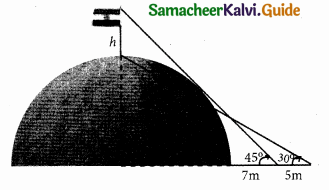Height of the Flag pole (ED) = h m
AC = (r + 7)
AB = (r + 7 + 5)
= (r + 12)
In the right ∆ ABD, tan 30° = $$\frac { AD }{ AB }$$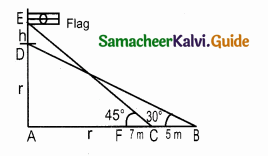$$\frac{1}{\sqrt{3}}=\frac{r}{r+12}$$
$$\sqrt { 3 }$$ r = r + 12
$$\sqrt { 3 }$$ r – r = 12 ⇒ r ($$\sqrt { 3 }$$ – 1) = 12
r[1.732 – 1] = 12 ⇒ 0.732 r = 12
r = $$\frac { 12 }{ 0.732 }$$ ⇒ = 16.39 m
In the right ∆ ACE, tan 45° = $$\frac { AE }{ AC }$$
1 + $$\frac { r+h }{ r+7 }$$
r + 7 = r + h
∴ h = 7 m
Height of the pole (h) = 7 m
Radius of the dome (r) = 16.39 mQuestion 6.
The top of a 15 m high tower makes an angle of elevation of 60° with the bottom of an electronic pole and angle of elevation of 30° with the top of the pole. What is the height of the electric pole?
Let the height of the electric pole AD be “h” m
EC = 15 – h m
Let AB be “x”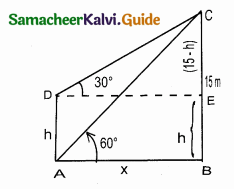In the right ∆ ABC, tan 60° = $$\frac { BC }{ AB }$$
$$\sqrt { 3 }$$ = $$\frac { 15 }{ x }$$
x = $$=\frac{15}{\sqrt{3}}=\frac{15 \times \sqrt{3}}{3}$$
= 5$$\sqrt { 3 }$$
In the right ∆ CDE, tan 30° = $$\frac { EC }{ DE }$$
$$\frac{1}{\sqrt{3}}=\frac{15-h}{x}$$ ………….(1)
Substitute the value of x = 5 $$\sqrt { 3 }$$ in (1)
$$\frac{1}{\sqrt{3}}=\frac{15-h}{5 \sqrt{3}} \Rightarrow \sqrt{3}(15-h)=5 \sqrt{3}$$
(15 – h) = $$\frac{5 \sqrt{3}}{\sqrt{3}}$$ ⇒ 15 – h = 5
h = 15 – 5 = 10
∴ Height of the electric pole = 10 m

Question 7.
A vertical pole fixed to the ground is divided in the ratio 1:9 by a mark on it with lower part shorter than the upper part. If the two parts subtend equal angles at a place on the ground, 25 m away from the base of the pole, what is the height of the pole?
Let the first part of the pole be “x” and the second part be “9x”
∴ height of the pole (AC) = x + 9x = 10x
Given ∠CDB = ∠BDA
∴ BD is the angle bisector of ∠ADC
By angle bisector theorem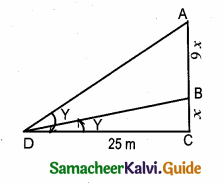$$\frac { AB }{ BC }$$ = $$\frac { AD }{ DC }$$
$$\frac { 9x }{ x }$$ = $$\frac { AD }{ 25 }$$ ⇒ AD = 9 × 25 = 225
In the right ∆ ACD
(225)2 = (10x)2 + 252
50625 = 100x2 + 625
∴ 100x2 = 50625 – 625 = 50000
x2 = $$\frac { 50000 }{ 100 }$$ = 500
x = $$\sqrt { 500 }$$ = $$\sqrt{5 \times 100}=10 \sqrt{5}$$
∴ AC = 10 × 10$$\sqrt { 5 }$$ = 100 $$\sqrt { 5 }$$ (AC = 10x)
∴ Height of the pole = 100 $$\sqrt { 5 }$$ mQuestion 8.
A traveler approaches a mountain on highway. He measures the angle of elevation to the peak at each milestone. At two consecutive milestones the angles measured are 4° and 8°. What is the height of the peak if the distance between consecutive milestones is 1 mile, (tan 4° = 0.0699, tan 8° = 0.1405)
Let the height of the peak be “h” mile. Let AD be x mile.
∴ AB = (x + 1) mile.
In the right ∆ ADC, tan 8° = $$\frac { AC }{ AC }$$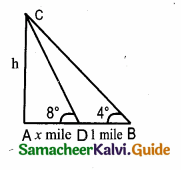0.1405 = $$\frac { h }{ x }$$
x = $$\frac { h }{ 0.1405 }$$ ………..(1)
In ∆ ABC, tan 4° = $$\frac { AC }{ AB }$$
0.0699 = $$\frac { h }{ x+1 }$$ ⇒ (x + 1) 0.0699 = h
0.0699x + 0.0699 = h
0.0699 x = h – 0.0699
x = $$\frac { h-0.0699 }{ 0.0699 }$$ ………(2)
Equation (1) and (2) we get,
$$\frac { h-0.0699 }{ 0.0699 }$$ = $$\frac { h }{ 0.1405 }$$
0.0699 h = 0.1405 (h – 0.0699)
0.0699 h = 0.1405 h – 0.0098
0.0098 = 0.1405 h – 0.0699 h
0.0098 = 0.0706 h
h = $$\frac { 0.0098 }{ 0.0706 }$$ = $$\frac { 98 }{ 706 }$$ = 0.1388
= 0.14 mile (approximately)
Height of the peak = 0.14 mile